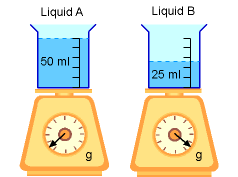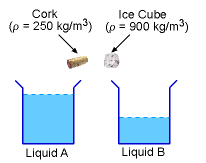Ch 1. Basics Multimedia Engineering Fluids MassDensity IdealGas Law Viscosity SurfaceTension VaporPressure
 Chapter 1. Basics 2. Fluid Statics 3. Kinematics 4. Laws (Integral) 5. Laws (Diff.) 6. Modeling/Similitude 7. Inviscid 8. Viscous 9. External Flow 10. Open-Channel Appendix Basic Math Units Basic Equations Water/Air Tables Sections Search eBooks Dynamics Fluids Math Mechanics Statics Thermodynamics Author(s): Chean Chin Ngo Kurt Gramoll ©Kurt GramollFLUID MECHANICS - CASE STUDY

IntroductionDensity MeasurementWill it Float or Sink?

High school students in a physics class are asked to measure the mass and volume of two unknown liquids. The raw data collected are summarized in the following table:

 Empty Container Mass (g) Container & Liquid Mass(g) Volume of Liquid (ml) Liquid A 25.0 75.0 50 Liquid B 25.0 44.7 25

Questions

Determine the densities of the two liquids, and identify the liquids. Also, predict if an ice cube and a cork will sink or float in the two liquids.

Approach

• The density of the ice cube is 900 kg/m3, and the density of the cork is 250 kg/m3.

Practice Homework and Test problems now available in the 'Eng Fluids' mobile app
Includes over 250 problems with complete detailed solutions.
Available now at the Google Play Store and Apple App Store.